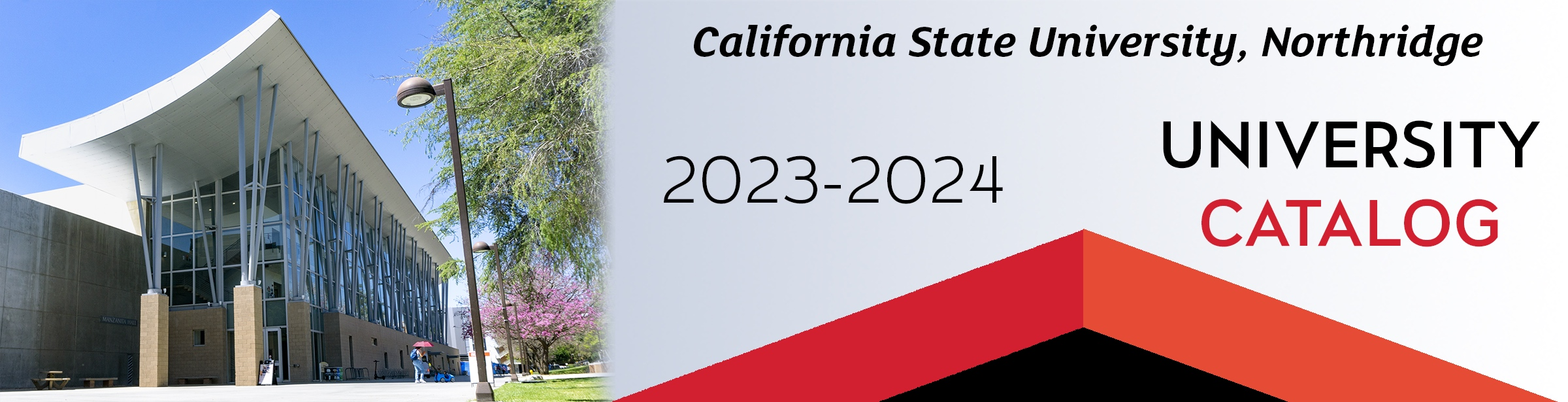## Course: MATH 105. Pre-Calculus II (5)

Prerequisites: Listed in Table 1. A preparation for the trigonometric, exponential, and logarithmic functions used in calculus. This course is intended for computer science, engineering, mathematics, and natural science majors. This course builds on student’s familiarity with exponential, logarithmic, and trigonometric expressions to achieve proficiency in analyzing the local and global behavior of functions involving such expressions. (Available for General Education, Basic Skills B4 Mathematics and Quantitative Reasoning.)

### MATH 105

Class NumberLocationDayTime